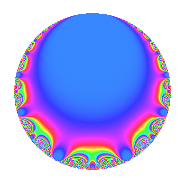# Properties

 Label 16.4.aLevel 16 Weight 4 Character orbit a Rep. character $$\chi_{16}(1,\cdot)$$ Character field $$\Q$$ Dimension 1 Newforms 1 Sturm bound 8 Trace bound 0

# Related objects

## Defining parameters

 Level: $$N$$ = $$16 = 2^{4}$$ Weight: $$k$$ = $$4$$ Character orbit: $$[\chi]$$ = 16.a (trivial) Character field: $$\Q$$ Newforms: $$1$$ Sturm bound: $$8$$ Trace bound: $$0$$

## Dimensions

The following table gives the dimensions of various subspaces of $$M_{4}(\Gamma_0(16))$$.

Total New Old
Modular forms 9 2 7
Cusp forms 3 1 2
Eisenstein series 6 1 5

The following table gives the dimensions of the cuspidal new subspaces with specified eigenvalues for the Atkin-Lehner operators and the Fricke involution.

$$2$$Dim.
$$+$$$$1$$

## Trace form

 $$q$$ $$\mathstrut +\mathstrut 4q^{3}$$ $$\mathstrut -\mathstrut 2q^{5}$$ $$\mathstrut -\mathstrut 24q^{7}$$ $$\mathstrut -\mathstrut 11q^{9}$$ $$\mathstrut +\mathstrut O(q^{10})$$ $$q$$ $$\mathstrut +\mathstrut 4q^{3}$$ $$\mathstrut -\mathstrut 2q^{5}$$ $$\mathstrut -\mathstrut 24q^{7}$$ $$\mathstrut -\mathstrut 11q^{9}$$ $$\mathstrut +\mathstrut 44q^{11}$$ $$\mathstrut +\mathstrut 22q^{13}$$ $$\mathstrut -\mathstrut 8q^{15}$$ $$\mathstrut +\mathstrut 50q^{17}$$ $$\mathstrut -\mathstrut 44q^{19}$$ $$\mathstrut -\mathstrut 96q^{21}$$ $$\mathstrut +\mathstrut 56q^{23}$$ $$\mathstrut -\mathstrut 121q^{25}$$ $$\mathstrut -\mathstrut 152q^{27}$$ $$\mathstrut +\mathstrut 198q^{29}$$ $$\mathstrut +\mathstrut 160q^{31}$$ $$\mathstrut +\mathstrut 176q^{33}$$ $$\mathstrut +\mathstrut 48q^{35}$$ $$\mathstrut -\mathstrut 162q^{37}$$ $$\mathstrut +\mathstrut 88q^{39}$$ $$\mathstrut -\mathstrut 198q^{41}$$ $$\mathstrut -\mathstrut 52q^{43}$$ $$\mathstrut +\mathstrut 22q^{45}$$ $$\mathstrut -\mathstrut 528q^{47}$$ $$\mathstrut +\mathstrut 233q^{49}$$ $$\mathstrut +\mathstrut 200q^{51}$$ $$\mathstrut -\mathstrut 242q^{53}$$ $$\mathstrut -\mathstrut 88q^{55}$$ $$\mathstrut -\mathstrut 176q^{57}$$ $$\mathstrut +\mathstrut 668q^{59}$$ $$\mathstrut +\mathstrut 550q^{61}$$ $$\mathstrut +\mathstrut 264q^{63}$$ $$\mathstrut -\mathstrut 44q^{65}$$ $$\mathstrut -\mathstrut 188q^{67}$$ $$\mathstrut +\mathstrut 224q^{69}$$ $$\mathstrut -\mathstrut 728q^{71}$$ $$\mathstrut +\mathstrut 154q^{73}$$ $$\mathstrut -\mathstrut 484q^{75}$$ $$\mathstrut -\mathstrut 1056q^{77}$$ $$\mathstrut +\mathstrut 656q^{79}$$ $$\mathstrut -\mathstrut 311q^{81}$$ $$\mathstrut -\mathstrut 236q^{83}$$ $$\mathstrut -\mathstrut 100q^{85}$$ $$\mathstrut +\mathstrut 792q^{87}$$ $$\mathstrut +\mathstrut 714q^{89}$$ $$\mathstrut -\mathstrut 528q^{91}$$ $$\mathstrut +\mathstrut 640q^{93}$$ $$\mathstrut +\mathstrut 88q^{95}$$ $$\mathstrut -\mathstrut 478q^{97}$$ $$\mathstrut -\mathstrut 484q^{99}$$ $$\mathstrut +\mathstrut O(q^{100})$$

## Decomposition of $$S_{4}^{\mathrm{new}}(\Gamma_0(16))$$ into irreducible Hecke orbits

Label Dim. $$A$$ Field CM Traces A-L signs $q$-expansion
$$a_2$$ $$a_3$$ $$a_5$$ $$a_7$$ 2
16.4.a.a $$1$$ $$0.944$$ $$\Q$$ None $$0$$ $$4$$ $$-2$$ $$-24$$ $$+$$ $$q+4q^{3}-2q^{5}-24q^{7}-11q^{9}+44q^{11}+\cdots$$

## Decomposition of $$S_{4}^{\mathrm{old}}(\Gamma_0(16))$$ into lower level spaces

$$S_{4}^{\mathrm{old}}(\Gamma_0(16)) \cong$$ $$S_{4}^{\mathrm{new}}(\Gamma_0(8))$$$$^{\oplus 2}$$